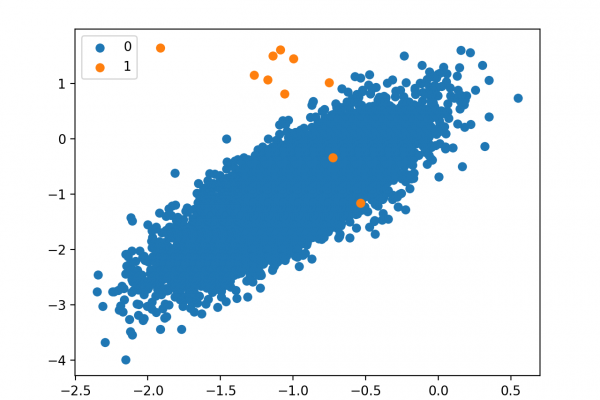# One-Class Classification Algorithms for Imbalanced DatasetsLast Updated on August 21, 2020

Outliers or anomalies are rare examples that do not fit in with the rest of the data.

Identifying outliers in data is referred to as outlier or anomaly detection and a subfield of machine learning focused on this problem is referred to as one-class classification. These are unsupervised learning algorithms that attempt to model “normal” examples in order to classify new examples as either normal or abnormal (e.g. outliers).

One-class classification algorithms can be used for binary classification tasks with a severely skewed class distribution. These techniques can be fit on the input examples from the majority class in the training dataset, then evaluated on a holdout test dataset.

Although not designed for these types of problems, one-class classification algorithms can be effective for imbalanced classification datasets where there are none or very few examples of the minority class, or datasets where there is no coherent structure to separate the classes that could be learned by a supervised algorithm.

In this tutorial, you will discover how to use one-class classification algorithms for datasets with severely skewed class distributions.

After completing this tutorial, you will know: